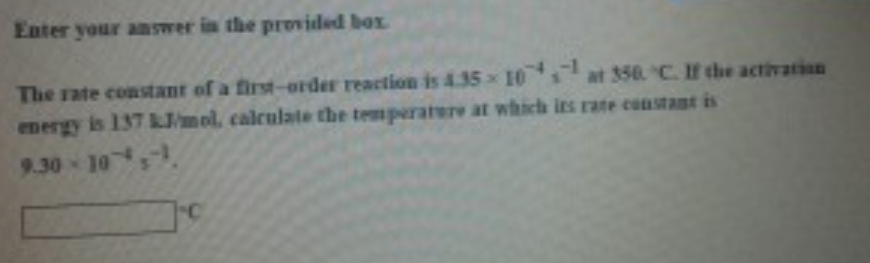# Problem: The rate constant of a first-order reaction is 4.35 times 10-4 s- at 350. °C. If the activation energy is 137 kJ/mol, calculate the temperature at which its rate constant is 9.30x10-4 S-1.

###### FREE Expert Solution
99% (294 ratings)###### Problem Details

The rate constant of a first-order reaction is 4.35 times 10-4 sat 350. °C. If the activation energy is 137 kJ/mol, calculate the temperature at which its rate constant is 9.30x10-4 S-1.